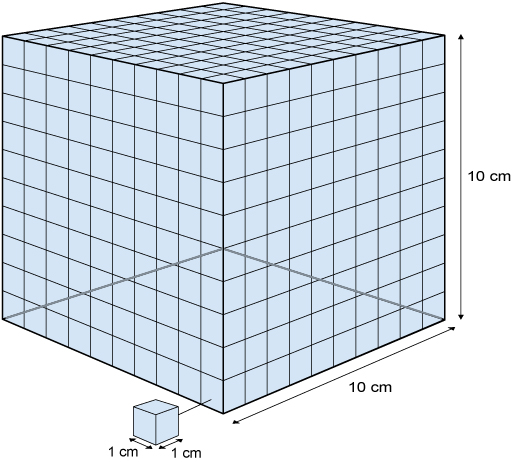Science, Maths & Technology

### Become an OU studentAn introduction to exoplanets

Start this free course now. Just create an account and sign in. Enrol and complete the course for a free statement of participation or digital badge if available.

# 4.2  Planet volumes

We live in a world of three dimensions, and volume describes how big something is in three dimensions. The volume is the total amount of space inside an object, or its capacity. People often use the letter V to represent volume. As you learned in Week 1, to qualify as a planet an object must be nearly spherical in shape, so to work out the volume of a planet you need to know the volume of a sphere.

You will start by looking at how to work out the volume of a cube, which is a much more straightforward calculation. Figure 8 shows two cubes: the smaller cube has sides of length 1 cm, while the larger cube has sides of length 10 cm.Figure _unit3.4.3 Figure 8  Two cubes: the smaller one with sides 1 cm × 1 cm × 1 cm, the larger one with sides 10 cm × 10 cm × 10 cm

The smaller cube has a volume of 1 cubic centimetre. 1 cubic centimetre is often written as 1 cm3. You can work out the volume of the larger cube by calculating how many small cubes you can fit into the larger one.

Look at one face of the large cube in Figure 8 and count the number of small cubes on that face. There are 100 (10 × 10) small cubes in a layer of the large cube. Each layer of 100 small cubes is stacked together to form the large cube, and there are 10 layers. So, there are 10 × 10 × 10 small cubes in the large cube. Since 10 × 10 × 10 = 1000 the volume of the large cube is therefore 1000 cm3.

If the length of the side of the cube is then the volume of the cube is . The volume of a cube is always the length of one of its edges cubed, that is, multiplied by itself three times.

The volume of a cube is easy to calculate, as you can see. You just need to multiply the length of one side by itself, then by itself again. This holds true, no matter how big the cube is. For a sphere, the volume is not just the size multiplied by itself three times. Because the shape is curved, the maths is more complicated, but there is a standard equation that uses the radius of a sphere to work out its volume. You may have encountered the exact equation before, but we can use a simplified version which is a good approximation:

V = 4.19r3

Here, just as with , r3 means r × r × r.

So, if you have a sphere with a radius of 1 m, then its volume will be 4.19 × 1 × 1 × 1 = 4.19 cubic metres, or 4.19 m3. Generally, volume is measured in m3 or cm3.